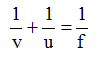Ex﻿

# 3. Light Reflection

#### Important Points To Be Remember (Light Reflection)

Important Points To Be Remember
Laws of reflection :
(a) Angle of incidence is equal to the angle of reflection .
(b) The incident ray, the reflected ray and the normal all lie in the same plane.
Mirror : A smooth, highly polished reflecting surface is called a mirror. There are two types of mirrors : (a) plane mirror (b) curved mirrors
Curved mirrors are classified as spherical mirrors or parabolic mirrors depending upon the curvature of the mirror.
Concave mirror : A spherical mirror whose inner hollow surface is the reflecting surface is called concave mirror. A concave mirror forms a real, inverted image of an object if the object is placed at any place outside F. However, when the object lies between F and P, the image formed is erect and virtual.
Convex mirror : A spherical mirror whose outer bulging at surface is the reflecting surface is called convex mirror. The image formed by a convex mirror is always erect, virtual and smaller than the object whatever may be the position of the object. A convex mirror is used as a side-mirror (driver’s mirror) on automobiles.
Aperture of a mirror : The effective width of a spherical mirror from which reflection can take place is called its aperture.
Pole : The centre of a curved mirror is called its pole.
Centre of curvature : The centre of the hollow sphere of which the spherical mirror is a part is called its centre of curvature. The centre of curvature of a concave mirror is in front of it, while that of a convex mirror is behind it.
Radius of curvature : Radius of the hollow sphere of which the mirror is a part is called its radius of curvature.
Principal axis : A straight line passing through the centre of curvature and pole of a spherical mirror is called its principal axis.
Focus : A point on the principal axis of a mirror at which the rays coming from infinity meet or appear to meet is called its focus. Focus is denoted by F.
Focal length : The distance between the pole of a spherical mirror and the focus is called the focal of a spherical mirror.
Real image : The image which can be obtained on a screen is called a real image. A real image is formed only when the reflected or refracted rays actually meet at a point.
Virtual image : The image which can be seen into a mirror but cannot be obtained on a screen is called a virtual image. A virtual image is formed only when the reflected or the refracted rays appear to come from a point.
Sign convention for spherical mirrors :
According to the sign convention for mirror, the focal length of a concave mirror is negative and that of a convex mirror is positive.
Mirrors formula : The relationship,is called the mirror formula.
Magnification : The ratio of the size of the image to that of the object is called magnification. For a mirror, magnification (M) is given by,Conclusions From The Sign Convention
For spherical mirror :
Table

If you want to give information about online courses to other students, then share it with more and more on Facebook, Twitter, Google Plus. The more the shares will be, the more students will benefit. The share buttons are given below for your convenience.
×

#### NTSE Physics (Class X)

• Electricity
• Magnetic Effect of Curent
• Light Reflection
• Light Refraction
• Human Eye & Colourful World
• Source of Energy
• Motion

#### NTSE Physics (Class IX)

• Force & laws of motion
• Work and Energy
• Gravitation
• Sound

SHOW CHAPTERS